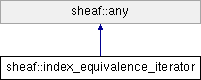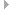SheafSystem  0.0.0.0
sheaf::index_equivalence_iterator Class Reference

An iterator over members of an id equivalence class. More...

`#include <index_equivalence_iterator.h>`

Inheritance diagram for sheaf::index_equivalence_iterator:## INDEX_EQUIVALENCE_ITERATOR FACET

typedef index_equivalence_class::member_type member_type
The member type for the equivalence id list. The first value in the pair is the index of the id space, the second value in the pair is the pod. More...

typedef index_equivalence_class::list_type::const_iterator iterator_type
The type of iterator for the equivalence id list. More...

const index_space_family_id_spaces
The id space family to iterate over. More...

pod_index_type _rep_id
The representative of this equivalence class. More...

bool _only_persistent
True, if only persistent id spaces should be iterated over. More...

bool _is_sorted
True, if the ids are sorted in increasing space id order. More...

index_equivalence_class _equivalence_class
The equivalence class to iterate over. More...

iterator_type _itr
The current equivalence list iterator. More...

iterator_type _end
The end of the current list begin iterated over. More...

member_type _item
The current equivalence class member. More...

bool _is_done
True if iteration is finished. More...

index_equivalence_iterator (const index_space_family &xid_spaces, pod_index_type xrep_id, bool xonly_persistent, bool xsort_ids)
Creates an instance for the id equivalence iterator of representative xrep_id in id space family xid_spaces. If xonly_persistent, only iterate over the persistent id spaces. If xsort_ids, sort the ids in increased space id order. More...

index_equivalence_iterator (const index_space_family &xid_spaces, const scoped_index &xrep_id, bool xonly_persistent, bool xsort_ids)
Creates an instance for the id equivalence iterator of representative xrep_id in id space family xid_spaces. If xonly_persistent, only iterate over the persistent id spaces. If xsort_ids, sort the ids in increased space id order. More...

index_equivalence_iterator (const index_space_family &xid_spaces, bool xonly_persistent, bool xsort_ids)
Creates an instance for the id equivalence iterator of invalid representative id in id space family xid_spaces. If xonly_persistent, only iterate over the persistent id spaces. If xsort_ids, sort the ids in increased space id order. More...

virtual ~index_equivalence_iterator ()
Destructor. More...

const index_space_familyid_spaces () const
The id space family of this equivalence class. More...

pod_index_type rep_id () const
The representative id in the top id space of this equivalence class. More...

void rep_id (scoped_index &result) const
The representative id of this equivalence class. More...

bool only_persistent () const
True, if only persistent id spaces should be iterated over. More...

bool is_sorted () const
True, if the ids are sorted in increasing space id order. More...

void put_rep_id (pod_index_type xrep_id)
Sets rep_id() to xrep_id. More...

void put_rep_id (const scoped_index &xrep_id)
Sets rep_id() to xrep_id. More...

const member_typeitem () const
The current equivalence class member. More...

bool is_done () const
True if iteration is finished. More...

size_type ct () const
The number of items in the current equivalence list being iterated over. More...

void next ()
Makes item() the next item in the equivalence class. More...

void reset ()
Restarts the iteration. More...

index_equivalence_iterator ()
Default constructor; disabled. More...

## ANY FACET

virtual bool is_ancestor_of (const any *other) const
Conformance test; true if other conforms to this. More...

virtual index_equivalence_iteratorclone () const
Virtual constructor, makes a new instance of the same type as this. More...

index_equivalence_iteratoroperator= (const index_equivalence_iterator &xother)
Assignment operator. More...

virtual bool invariant () const
Class invariant. More...Public Member Functions inherited from sheaf::any
bool is_same_type (const any *other) const
True if other is the same type as this. More...

virtual ~any ()
Destructor. More...

bool invariant_check () const
True if invariant checking is enabled. More...

void enable_invariant_check () const
Enable invariant checking. More...

void disable_invariant_check () const
Disable invariant check. Intended for preventing recursive calls to invariant and for suppressing invariant checking during multi-phase initialization. More...

int disable_invariance_check_request_depth () const
Number of times disable_invariant_check has been called without matching call to enable_invariant_check. More...Protected Member Functions inherited from sheaf::any
any ()
default constructor More...

## Detailed Description

An iterator over members of an id equivalence class.

Definition at line 48 of file index_equivalence_iterator.h.

## ◆ iterator_type

 typedef index_equivalence_class::list_type::const_iterator sheaf::index_equivalence_iterator::iterator_type
protected

The type of iterator for the equivalence id list.

Definition at line 167 of file index_equivalence_iterator.h.

## ◆ member_type

The member type for the equivalence id list. The first value in the pair is the index of the id space, the second value in the pair is the pod.

Definition at line 63 of file index_equivalence_iterator.h.

## ◆ index_equivalence_iterator() [1/4]

 sheaf::index_equivalence_iterator::index_equivalence_iterator ( const index_space_family & xid_spaces, pod_index_type xrep_id, bool xonly_persistent, bool xsort_ids )

Creates an instance for the id equivalence iterator of representative xrep_id in id space family xid_spaces. If xonly_persistent, only iterate over the persistent id spaces. If xsort_ids, sort the ids in increased space id order.

Precondition
• is_valid(xrep_id) ? xid_spaces.hub_id_space().contains(xrep_id) : true
Postcondition

Definition at line 33 of file index_equivalence_iterator.cc.

## ◆ index_equivalence_iterator() [2/4]

 sheaf::index_equivalence_iterator::index_equivalence_iterator ( const index_space_family & xid_spaces, const scoped_index & xrep_id, bool xonly_persistent, bool xsort_ids )

Creates an instance for the id equivalence iterator of representative xrep_id in id space family xid_spaces. If xonly_persistent, only iterate over the persistent id spaces. If xsort_ids, sort the ids in increased space id order.

Precondition
• xrep_id.is_valid() ? xid_spaces.hub_id_space().contains(xrep_id.hub_pod()) : true
Postcondition

Definition at line 66 of file index_equivalence_iterator.cc.

## ◆ index_equivalence_iterator() [3/4]

 sheaf::index_equivalence_iterator::index_equivalence_iterator ( const index_space_family & xid_spaces, bool xonly_persistent, bool xsort_ids )

Creates an instance for the id equivalence iterator of invalid representative id in id space family xid_spaces. If xonly_persistent, only iterate over the persistent id spaces. If xsort_ids, sort the ids in increased space id order.

Postcondition

Definition at line 99 of file index_equivalence_iterator.cc.

## ◆ ~index_equivalence_iterator()

 sheaf::index_equivalence_iterator::~index_equivalence_iterator ( )
virtual

Destructor.

Definition at line 128 of file index_equivalence_iterator.cc.

References id_spaces().

Referenced by index_equivalence_iterator().

## ◆ index_equivalence_iterator() [4/4]

 sheaf::index_equivalence_iterator::index_equivalence_iterator ( )
inlineprotected

Default constructor; disabled.

Definition at line 172 of file index_equivalence_iterator.h.

Referenced by clone(), and index_equivalence_iterator().

## ◆ clone()

 sheaf::index_equivalence_iterator * sheaf::index_equivalence_iterator::clone ( ) const
virtual

Virtual constructor, makes a new instance of the same type as this.

Postcondition
• result != 0
• is_same_type(result)

Reimplemented from sheaf::any.

Definition at line 400 of file index_equivalence_iterator.cc.

Referenced by is_ancestor_of().

## ◆ ct()

 sheaf::size_type sheaf::index_equivalence_iterator::ct ( ) const

The number of items in the current equivalence list being iterated over.

Postcondition
• result >= 0

Definition at line 259 of file index_equivalence_iterator.cc.

Referenced by sheaf::member_record::external_size(), and is_done().

## ◆ id_spaces()

 const sheaf::index_space_family & sheaf::index_equivalence_iterator::id_spaces ( ) const

The id space family of this equivalence class.

Definition at line 145 of file index_equivalence_iterator.cc.

References _id_spaces, and rep_id().

Referenced by clone(), index_equivalence_iterator(), invariant(), put_rep_id(), and ~index_equivalence_iterator().

## ◆ invariant()

 bool sheaf::index_equivalence_iterator::invariant ( ) const
virtual

Class invariant.

Invariant
Hack:
section_space_schema_poset internal_id hack. !rep_id().is_scoped should go away once the section_space_schema_poset has a scoped internal_id.
Invariant
• is_valid(rep_id()) ? id_spaces().hub_id_space().contains(rep_id()) : true

Reimplemented from sheaf::any.

Definition at line 455 of file index_equivalence_iterator.cc.

Referenced by index_equivalence_iterator(), next(), operator=(), put_rep_id(), and reset().

## ◆ is_ancestor_of()

 bool sheaf::index_equivalence_iterator::is_ancestor_of ( const any * other ) const
virtual

Conformance test; true if other conforms to this.

Precondition
• other != 0

Reimplemented from sheaf::any.

Definition at line 379 of file index_equivalence_iterator.cc.

References clone().

Referenced by reset().

## ◆ is_done()

 bool sheaf::index_equivalence_iterator::is_done ( ) const

True if iteration is finished.

Postcondition
• is_basic_query

Definition at line 240 of file index_equivalence_iterator.cc.

References _is_done, and ct().

Referenced by item(), next(), and sheaf::operator<<().

## ◆ is_sorted()

 bool sheaf::index_equivalence_iterator::is_sorted ( ) const

True, if the ids are sorted in increasing space id order.

Definition at line 173 of file index_equivalence_iterator.cc.

References _is_sorted, and put_rep_id().

Referenced by clone(), index_equivalence_iterator(), and only_persistent().

## ◆ item()

 const sheaf::index_equivalence_iterator::member_type & sheaf::index_equivalence_iterator::item ( ) const

The current equivalence class member.

Precondition
• !is_done()

Definition at line 221 of file index_equivalence_iterator.cc.

References _item, and is_done().

Referenced by next(), sheaf::operator<<(), and put_rep_id().

## ◆ next()

 void sheaf::index_equivalence_iterator::next ( )

Makes item() the next item in the equivalence class.

Precondition
• !is_done()
Postcondition
• !is_done() ? item().first != old_space_index : true

Definition at line 280 of file index_equivalence_iterator.cc.

References _end, _is_done, _item, _itr, invariant(), is_done(), item(), and reset().

Referenced by ct(), and sheaf::operator<<().

## ◆ only_persistent()

 bool sheaf::index_equivalence_iterator::only_persistent ( ) const

True, if only persistent id spaces should be iterated over.

Definition at line 166 of file index_equivalence_iterator.cc.

References _only_persistent, and is_sorted().

Referenced by clone(), index_equivalence_iterator(), and rep_id().

## ◆ operator=()

 sheaf::index_equivalence_iterator & sheaf::index_equivalence_iterator::operator= ( const index_equivalence_iterator & xother )

Assignment operator.

Postcondition

Definition at line 428 of file index_equivalence_iterator.cc.

References _end, _equivalence_class, _id_spaces, _is_done, _is_sorted, _item, _itr, _only_persistent, _rep_id, and invariant().

Referenced by clone().

## ◆ put_rep_id() [1/2]

 void sheaf::index_equivalence_iterator::put_rep_id ( pod_index_type xrep_id )

Sets rep_id() to xrep_id.

Postcondition

Definition at line 180 of file index_equivalence_iterator.cc.

References _rep_id, invariant(), and rep_id().

Referenced by sheaf::member_record::has_equivalence_iterator(), is_sorted(), and sheaf::operator<<().

## ◆ put_rep_id() [2/2]

 void sheaf::index_equivalence_iterator::put_rep_id ( const scoped_index & xrep_id )

Sets rep_id() to xrep_id.

Precondition
• xrep_id.is_valid() ? id_spaces().hub_id_space().contains(xrep_id.hub_pod()) : true
Postcondition

Definition at line 200 of file index_equivalence_iterator.cc.

## ◆ rep_id() [1/2]

 sheaf::pod_index_type sheaf::index_equivalence_iterator::rep_id ( ) const

The representative id in the top id space of this equivalence class.

Definition at line 152 of file index_equivalence_iterator.cc.

References _rep_id.

## ◆ rep_id() [2/2]

 void sheaf::index_equivalence_iterator::rep_id ( scoped_index & result ) const

The representative id of this equivalence class.

Definition at line 159 of file index_equivalence_iterator.cc.

## ◆ reset()

 void sheaf::index_equivalence_iterator::reset ( )

Restarts the iteration.

Postcondition

Definition at line 320 of file index_equivalence_iterator.cc.

## ◆ _end

 iterator_type sheaf::index_equivalence_iterator::_end
protected

The end of the current list begin iterated over.

Definition at line 207 of file index_equivalence_iterator.h.

Referenced by next(), operator=(), and reset().

## ◆ _equivalence_class

 index_equivalence_class sheaf::index_equivalence_iterator::_equivalence_class
protected

The equivalence class to iterate over.

Definition at line 197 of file index_equivalence_iterator.h.

Referenced by ct(), operator=(), and reset().

## ◆ _id_spaces

 const index_space_family* sheaf::index_equivalence_iterator::_id_spaces
protected

The id space family to iterate over.

Definition at line 172 of file index_equivalence_iterator.h.

Referenced by clone(), id_spaces(), index_equivalence_iterator(), operator=(), and rep_id().

## ◆ _is_done

 bool sheaf::index_equivalence_iterator::_is_done
protected

True if iteration is finished.

Definition at line 217 of file index_equivalence_iterator.h.

Referenced by is_done(), next(), operator=(), and reset().

## ◆ _is_sorted

 bool sheaf::index_equivalence_iterator::_is_sorted
protected

True, if the ids are sorted in increasing space id order.

Definition at line 192 of file index_equivalence_iterator.h.

Referenced by clone(), index_equivalence_iterator(), is_sorted(), and operator=().

## ◆ _item

 member_type sheaf::index_equivalence_iterator::_item
protected

The current equivalence class member.

Definition at line 212 of file index_equivalence_iterator.h.

Referenced by item(), next(), operator=(), and reset().

## ◆ _itr

 iterator_type sheaf::index_equivalence_iterator::_itr
protected

The current equivalence list iterator.

Definition at line 202 of file index_equivalence_iterator.h.

Referenced by next(), operator=(), and reset().

## ◆ _only_persistent

 bool sheaf::index_equivalence_iterator::_only_persistent
protected

True, if only persistent id spaces should be iterated over.

Definition at line 187 of file index_equivalence_iterator.h.

Referenced by clone(), index_equivalence_iterator(), only_persistent(), and operator=().

## ◆ _rep_id

 pod_index_type sheaf::index_equivalence_iterator::_rep_id
protected

The representative of this equivalence class.

Definition at line 182 of file index_equivalence_iterator.h.

Referenced by clone(), ct(), index_equivalence_iterator(), operator=(), put_rep_id(), rep_id(), and reset().

The documentation for this class was generated from the following files: